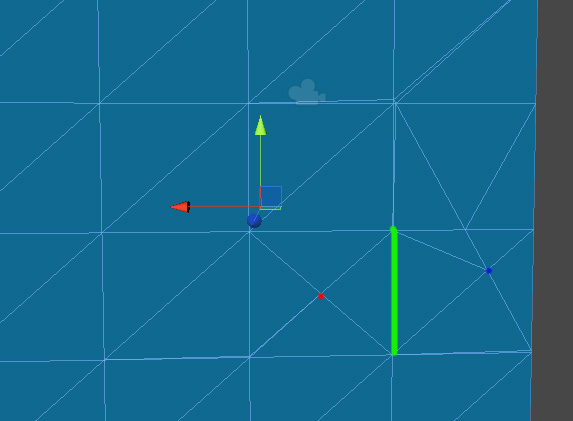# Connect 2 verticies

Hi there! A need to connect red and blue vertecies, by creating additional triangles.So first thing I do, is get their positions; p1r, p2b
Then, I calculate direction vector; _directin = p2b - p1r

Next step, I need to create new vertex in that edge I marked as green.
I here I’m clueless how to proced.

Red vertex shares 4 triangles, I can get them, but I need to:

1. Determine, which triangle I need to use
2. And find new vertex position on the edge which is closes to the other blue vertex

And advice on how should I handle it?

I though of comparing vertices by their world positions, then get edge world position, somehow interpolate where new vertex should be put, then create it using TransformInverse, but maybe I can do it more efficiently, using some clever trigonometry or other solution I’m not aware of.

Well, if it’s a fully shared mesh it’s very easy. Just determine all triangles which use the red vertex and the blue vertex. The red has 4 triangles the blue has 5. Just iterate through the red triangles and compare the other two vertices for each triangle with the outer vertices of the blue triangles. There are exact 3 triangle pairs which share at least one vertex with each other. Just pick the one where both outer vertices match.

This can be done entirely with the triangle list. So just compare the integer values.

If the vertices are not shared it’s getting mor complicated. You have to compare them on a position base since neighbor triangles aren’t connected. Never direct compare positions, always use an approximation.

``````// C#